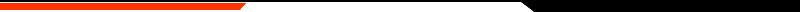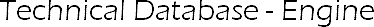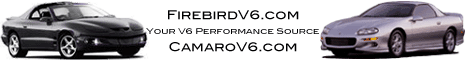Main  -  Message Board  -  Technical Database  -  Engine Tech  -  Modifications Encyclopedia  -  Media  -  Timeslips  -  Store  -  Events### Formulas/ Calculations

#### Air Filters

The amount of horsepower an engine makes is directly related to the mass airflow of the engine. Mass airflow is determined by volumetric efficiency VE, and the density (pressure-and-temperature) of the air. In turn, if you know the actual efficiency of the engine, and energy content of the fuel used, you can predict horsepower.

Assume a  97.5% VE at 5400. At standard temperature and pressure (77 degrees Fahrenheit, and 14.53 psi) this equals 22.56 lbs/hour air. Assuming 19000 BTUs per gallon of gas and a 0.33 (mechanical x thermal) efficiency this should produce 228 hp.  Converting the mass flow to CFM = 309CFM. Now, add a K&N cone filter into the picture.  It may positively impact VE due to a lower pressure drop at 309 CFM than the stock filter. Let’s say VE goes to 98%.

Based on the same calculation, the mass airflow is now 22.68 lbs/hr or230 HP. However, consider the temperature of the air. Under the hood, without any type of insulation, the temperature is probably not far from the temp of the thermostat. To be conservative, let’s say the new cone filter is breathing in 160 degree under hood air instead of 77 degree air. Using the previous formula, but adjusting the air temperature what is the mass flow rate? 19.64 lbs/hour. That amounts to 199 horsepower. Here are calcs for a couple other temps at 98% VE:

 Temp Mass Air Flow Predicted HP 40 24.36 247 77 22.68 230 100 21.75 220 120 21.0 213 140 20.3 206 160 19.64 199 180 19.03 193

#### Throttle Body Sizes

(All sizes have been converted to cross-sectional area per liter of displacement for comparison)

LT1 (5.7 liter)

Stock 2x48mm (68mm) = 3619mm2 / 5.7 = 643mm2 per liter

Big 2x52mm (74mm) = 4247mm2 / 5.7 = 745mm2 per liter

Bigger 2x58mm (82mm) = 5284mm2 / 5.7 = 927mm2 per liter

L36 (3.8 liter)

Stock 65mm = 3318mm2 / 3.8 = 873mm2 per liter

Mustang 3.8L

2000 Stock 60mm TB, and 70mm MAF

1998 Stock TB is 50mm, a stock MAF is 70mm

1995 Stock TB size is 50mm

#### Airflow vs HP

Engine airflow can be directly related to hp developed. The volumetric efficiency of an engine is always the highest at its torque peak. Above this rpm, pumping efficiency drops off. A round average for airflow vs. hp is 1.5 to 1.6 cubic feet per minute for each hp developed. If you want to work this out in mass flow, a standard cubic foot of air weighs about 0.0765 pounds on a standard 15C day. For example if we have a 4 cylinder engine developing 200hp it would be flowing around 300 SCFM or 23 lbs./min. using the 1.5 figure.  In CFH and lbs./hr. we would have 18,000 and 1380 respectively. It should be noted that the density of air varies with its temperature. A cubic foot of cold air weighs more than a cubic foot of hot air.

#### BSFC

The brake specific fuel consumption of an engine depends on many factors including thermal efficiency, mechanical efficiency and air to fuel ratios. Most piston engines have a BSFC of between 0.5 and 0.55 lbs. of fuel per hp per hour at maximum power, set fairly rich with AFRs between 12 and 13 to 1. Using a BSFC of 0.5, our hypothetical 200 hp engine would use about 100 pounds of fuel per hour. We can work back from airflow and determine that the actual fuel to air ratio was 13.8 to one.

#### Fuel Injector Duty Cycle

Duty cycle refers to the amount of time an injector is held open vs. the amount of time available at a certain rpm before the next injection event happens and is expressed as a percentage. The electrical characteristics of injectors make it undesirable to drive them at 100% duty cycle due to heat distress on the injector windings and drive circuitry. We like to limit maximum duty cycle to a value between 70 and 85% for most applications. If we take our figure of 400 hp and multiply it by 0.8, we get a maximum figure of 320 hp. Working back, we can determine that the injector duty cycle should only be about 50% on our example engine with these injectors, so we are very safe here.

We can calculate hp and fuel consumption from duty cycle directly using our assumptions above and can even develop rough hp and torque curves using the DUTY readout from your SDS programmer.

At 3000 rpm and full throttle, we stabilize rpm momentarily by using the brakes. Wait 2 seconds for any acceleration enrichment to stop which affects duty cycle. Let's say that the duty cycle reads 15% on our example setup. We need to take our 400 hp figure and multiply by 0.15. This gives us 60 hp. Working back, we can figure that we are burning about 30 lbs./hr under these conditions. This can be converted to gallons per hour and even MPG if velocity is known. This procedure can be used at 500 rpm intervals to develop a hp curve.

Torque output can be calculated from hp by using: Torque = 5252 times hp divided by rpm. Conversely, if torque is known from a dyno test, you can play with the numbers and calculate hp using HP=torque times rpm divided by 5252. In our example, torque works out to 105 ft./lbs. It should be noted that most properly mapped engines will run at a lower BSFC figure during cruise conditions because of the leaner AFRs generally used here. You could use a BSFC figure of 0.38 to 0.45 here if the AFRs are in the 16 to 17 to 1 range as indicated on your mixture meter.

#### Fuel Mass

The weight or mass of fuel also varies with temperature and its molecular weight. Gasolines with high aromatic concentrations weigh more per unit volume than regular pump fuel. As a round figure, gasoline weighs about 7.2lbs. per imperial gallon or 6 lbs. per gallon or 1.586 lbs. per liter and 0.001586lbs./cc at 15C. To convert an injector flow rate from lbs. per hour(X)to lbs./min.(Y), we can deduce that X divided by 60 will give us lbs./min.(Y). Y divided by 0.001586 will give us ccs per minute(Z). As an example, we have a 50 lb./hr. injector. 50 divided by 60equals 0.833. 0.833 divided by 0.001586 equals 525. So as a rough guide we can say that multiplying and dividing injector flow rates in the 2 popular flow units by 10 gives us a close approximation conversion. A 500cc injector is about a 50 lb. injector.

The number of injectors multiplied by the flowrate gives the maximum amount of fuel that can be delivered to the engine and determines the maximum hp that can be developed. Using what we have learned above, we can determine that with our four, 50 lb. injectors we can flow 200 lbs. of fuel per hour and that we have enough fuel flow capacity to develop 400 hp at a BSFC of 0.5 lbs/hp/hr. at 100%injector duty cycle.

#### Fuel Injector Sizing

To select a proper injector for your application you need to know the intended peak horsepower and of course the number of injectors in the system.  The Brake Specific Fuel Consumption (BSFC) figure of .5 lb fuel/hp-hour is a well agreed upon value for most engines.

For example on a 300hp 8 injector system: 300hp x .5 lb fuel/hp-hr: 150 lbs fuel/hr. 150 lbsfuel/8 injectors : 18.75 lb/hr injectors @ l00% duty cycle.

Assuming a correction factor at 75% duty cycle (75-80% is optimal for Fuel Injected engines), as you should never hold the injectors wide open or 'static'.  To calculate the injector size divide the calculated static figure of 18.75 lb/hr by .75. This gives you 25 lbs/hr or a 25 lb/hr injector.  In this application your injectors would be using a 75% duty cycle to maintain a constant 300 hp.

Assuming 80%
lb/hr = (HP * .5) / (num. injectors * .8)

to solve for hp, you can reverse it :
HP = (lb/hr * num. injectors * 0.8) / 0.5

so, say we take the hp of the 3.4L, that would come out to 160. so we solve to see what size (lb\hr) injectors we need:

lb\hr = (160 * .5) / (6 * .8)
lb\hr = (80) / (4.8)
lb\hr = 16.67

so basically the 17 lb\hr injectors on the 3.4L car are a bit more than actually needed, but not much.

So then we solve for the 3.8L:

lb\hr = (200 * .5) / (6 * .8)
lb\hr = (100) / (4.8)
lb\hr = 20.83

Stock injectors on a 3.8L are 22 lbs/hr.

So now we solve for a modified 300HP3.8L:

lb\hr = (300 * .5) / (6 * .8)
lb\hr = (150) / (4.8)
lb\hr = 31.25

So the injectors on a 3.8 to support 300HP could use the L67 36 lbs/hr.